# Intermediate Algebra Tutorial 19

Intermediate Algebra
Tutorial 19: Solving Systems of Linear Equations
in Two VariablesAnswer/Discussion to 1a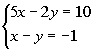Step 1: Graph the first equation.

x-intercept*Plug in 0 for y for x-int

*Inverse of mult. by 5 is div. by 5

*x-intercept

The x-intercept is (2, 0).

y-intercept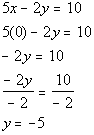*Plug in 0 for x for y-int

*Inverse of mult. by -2 is div. by -2

*y-intercept

The y-intercept is (0, -5).

Find another solution by letting x = 1.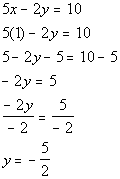*Plug in 1 for x
*Inverse of add 5 is sub. 5

*Inverse of mult. by -2 is div. by -2

Another solution is (1, -5/2).

Solutions:

x y (x, y) 2 0 (2, 0) 0 -5 (0, -5) 1 -5/2 (1, -5/2)

Plotting the ordered pair solutions and drawing the line: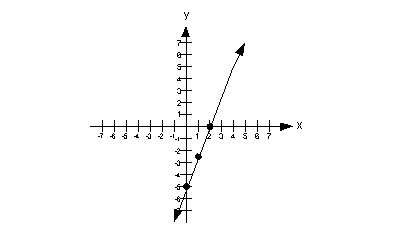Step 2: Graph the second equation on the same coordinate system as the first.

x-intercept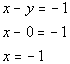*Plug in 0 for y for x-int
*x-intercept

The x-intercept is (-1, 0).

y-intercept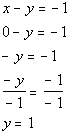*Plug in 0 for x for y-int

*Inverse of mult. by -1 is div. by -1

*y-intercept

The y-intercept is (0, 1).

Find another solution by letting x = 1.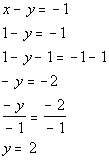*Plug in 1 for x
*Inverse of add 1 is sub. 1

*Inverse of mult. by -1 is div by -1

Another solution is (1, 2).

Solutions:
x y (x, y) -1 0 (-1, 0) 0 1 (0, 1) 1 2 (1, 2)

Plotting the ordered pair solutions and drawing the line: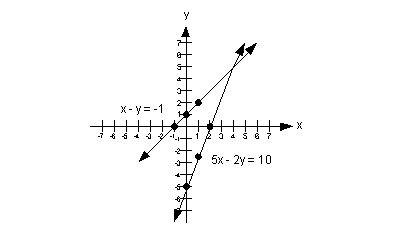Step 3: Find the solution.

We need to ask ourselves, is there any place that the two lines intersect, and if so, where?

The answer is yes, they intersect at (4, 5).

Step 4: Check the proposed ordered pair solution in BOTH equations.

You will find that if you plug the ordered pair (4, 5) into BOTH equations of the original system, that this is a solution to BOTH of them.

The solution to this system is (4, 5).Answer/Discussion to 2a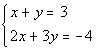Step 1: Simplify if needed.

Both of these equations are already simplified.  No work needs to be done here.

Step 2:  Solve one equation for either variable.

Neither equation is already solved for either variable.  It looks like equation one is the easiest one to work with.  Either x or y would be fine to solve for in the first equation since they both have a 1 as a coefficient.

I will choose to solve for x in the first equation:
(Note it would be perfectly fine to solve for y)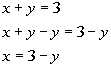*Inverse of add y is sub. y
*Solved for x

Substitute the expression 3 - y for x into the second equation and solve for y:
(when you plug in an expression like this, it is just like you plug in a number for your variable)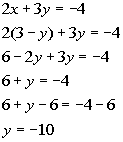*Sub. 3 - y in for x
*Dist. 2 through (  )
*Combine like terms

*Inverse of add 6 is sub. 6

Step 5:  Solve for second variable.

Plug in -10 for y into the equation in step 2 to find x's value.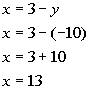*Plug in -10 for y

Step 6: Check the proposed ordered pair solution in BOTH original equations.

You will find that if you plug the ordered pair (13, -10) into BOTH equations of the original system, that this is a solution to BOTH of them.

(13, -10) is a solution to our system.Answer/Discussion to 3a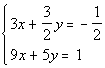Step 1: Simplify and put both equations in the form Ax + By = C if needed.

Since we have some fractions in this problem, let's go ahead and get rid of them to simplify things.

Multiplying first equation by it's LCD we get: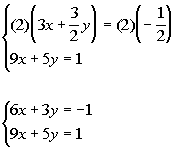*Mult. by LCD of 2

Step 2: Multiply one or both equations by a number that will create opposite coefficients for either x or y if needed.

We can either work with the x's or the y's; it doesn't matter.

I'm going to choose to work with the x's. The smallest number that both 6 and 9  go into is 18.  This works in the same fashion as LCD, you want the smallest number they both go into.  Make one of them positive and the other negative (it doesn't matter which one is which), so that you have opposites.

Multiplying the first equation by 3 and the second equation by -2 we get: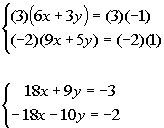*Mult. both sides of 1st eq. by 3
*Mult. both sides of 2nd eq. by -2

*x's have opposite coefficients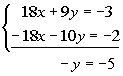*Note that x's dropped out

Step 4: Solve for remaining variable.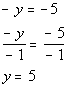*Inverse of mult. by -1 is div. by -1

Step 5: Solve for second variable.

You can choose any equation used in this problem to plug in the found y value.

I choose to plug in 5 for y into the second equation to find x's value.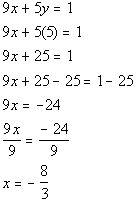*Plug in 5 for y

*Inverse of add 25 is sub. 25

*Inverse of mult. by 9 is div. by 9

Step 6: Check the proposed ordered pair solution in BOTH original equations.

You will find that if you plug the ordered pair (-8/3, 5) into BOTH equations of the original system, that this is a solution to BOTH of them.

(-8/3, 5) is a solution to our system.

Last revised on July 10, 2011 by Kim Seward.